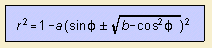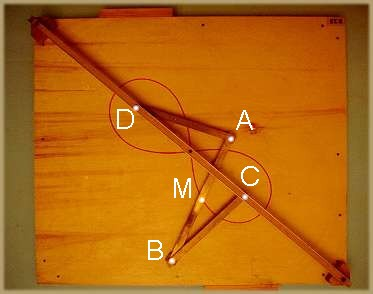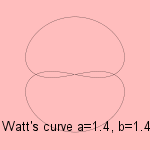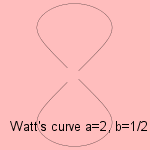Watt's curve

sexticwhere both a and b > 0.

James Watt (1736-1819) was the guy who developed the steam engine. From the movement of the wheels of the locomotives he derived the Watt's curve.The curve describes the path of the midpoints of a rotating rod: given two wheels (e.g. of a steam locomotive) of radius R (AD=BC in picture), having their centers a distance d (CD) from each other. A rod of length l (AB) is fixed at each end to the circumference of the two rotating wheels. Then the mid-point (M) of the rod describes the path of a Watt's curve 1.

The curve is a tricircular algebraic curve.The octic Cartesian equation following from the polar equation above 2) can we simplified to a sextic equation 3).
When the length of the rod and the distance between the circle's centers are equal (i.e. b=1), the curve is a hippopede.
In this case, for parameter 'a' having the value 1/2 (the length of the rod is √2 times the radius of the circle) a lemniscate occurs (surrounded by a circle).

A Watt's curve describes a linkage. Mathematicians like Sylvester, Kempe and Cayley worked on the theory of linkages in the 1870's. It can be proved that every finite segment of an algebraic curve can be generated by a linkage.

notes

1) Parameters a and b can be expressed in the given distances R, d and l as follows:

• a = d2 / 4 R2
• b = l2 / d2

2) In  Cartesian coordinates:

4y2((x2+y2)b2-x2)=((x2+y2)(x2+y2-a2+b2)-x2+y2)2

3) Sextic Cartesian equation:

(x2+y2)3-2(x2+y2)2+(x+4y2)(x2+y2)=dy2